# Venn Diagrams

The Venn diagrams are basically used to solve the questions related to syllogism easily. As the syllogisms is an important part of the logical reasoning section, it is very crucial to be well-versed with the venn diagrams for CAT topic to be able to solve the related questions effectively.

## Venn Diagram: Definition

A Venn diagram can be defined as an illustration of the relationships between and among sets and/or groups of objects that share something in common. Usually, Venn diagrams are used to depict set intersections as given below.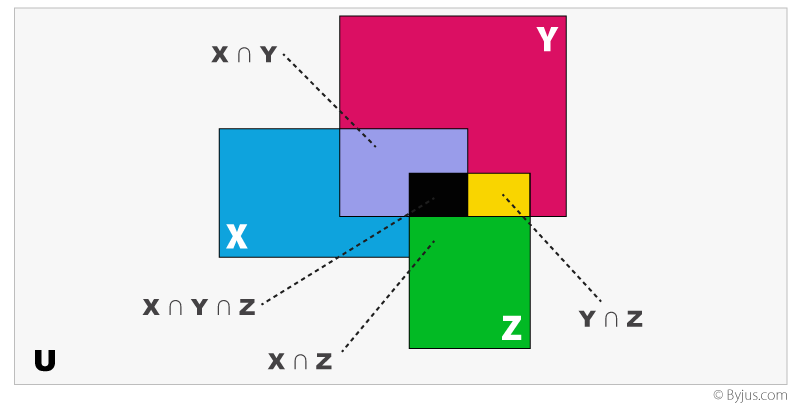The drawing is an example of a Venn diagram that shows the relationship among three overlapping sets X, Y, and Z.
There are some regions which are common to X & Y(X ∩Y), X & Z (X ∩Z),Y & Z (Y ∩Z) and all three of X,Y,Z (X ∩Y ∩Z). These are called intersecting regions.

#### A typical three-set Venn diagram can be represented as follows: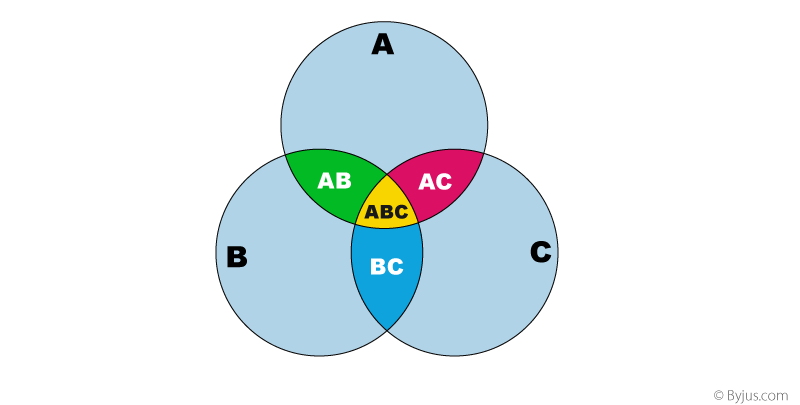### Some important Venn Diagrams:

• #### All A are B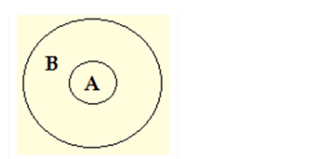This implies that A is a subset of B i.e. A is encompassed in B but not necessarily vice versa.

• #### A = B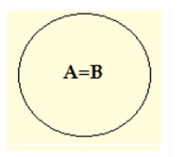This implies that both A and B are subsets of each other A i.e. both the A and B are contained in each other.

• #### No A are B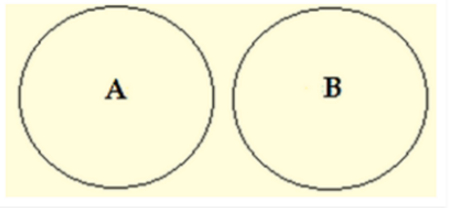This implies that both A and B are not contained in each other i.e. it can be said that both A and B are independent sets.

• #### Some A are B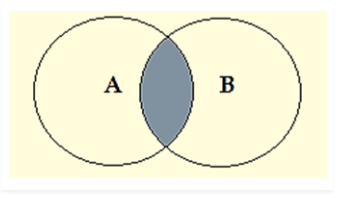Here some part of A and some part of B are intersecting and also, some B areA also holds true.

• #### Some A are not B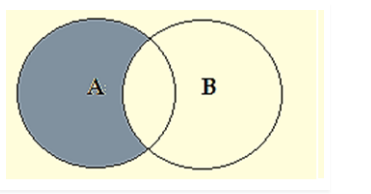Here, this implies that some part of A is surely not included in B and it is uncertain whether the remaining part of A is included in B or not.

To learn various other CAT important topics, stay tuned with Byju’s. Also, get various engaging video lessons, CAT Sample Papers, and previous year question papers to prepare for the CAT exam more effectively.